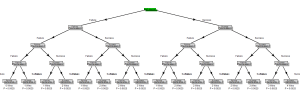## Edimax EW-7811Un USB wireless – connecting to a network (on ubuntu 11.10)

I recently decided to make the plunge and install ubuntu 11.10 (32 bit) on my desktop. All went smoothly except for one bug: I couldn’t get Internet.

I use a wireless USB stick by edimax (it is called IEEE802.11b/g/n nano USB adapter or also EW-7811Un). The problem was that Ubuntu seems to be able to use the USB to see the networks around me, but when I tried to connect to my network (either when the router had the password on or off) – it just kept trying and failing to connect.

This is apparently a known bug which can be resolved after following some good leads from ubuntuforums (thanks the user “praseodym” for your help) and askubuntu (thank you user Engels Peralta for your help).

Bellow are the steps I needed to take in order to solve the problem in the smoothest fashion – I hope others might benefit from it in the future.

## Diagram for a Bernoulli process (using R)

A Bernoulli process is a sequence of Bernoulli trials (the realization of n binary random variables), taking two values (0/1, Heads/Tails, Boy/Girl, etc…). It is often used in teaching introductory probability/statistics classes about the binomial distribution. When visualizing a Bernoulli process, it is common to use a binary tree diagram in order to show the […]

A Bernoulli process is a sequence of Bernoulli trials (the realization of n binary random variables), taking two values (0/1, Heads/Tails, Boy/Girl, etc…). It is often used in teaching introductory probability/statistics classes about the binomial distribution.

When visualizing a Bernoulli process, it is common to use a binary tree diagram in order to show the progression of the process, as well as the various consequences of the trial. We might also include the number of “successes”, and the probability for reaching a specific terminal node.

I wanted to be able to create such a diagram using R. For this purpose I composed some code which uses the {diagram} R package. The final function should allow one to create different sizes of diagrams, while allowing flexibility with regards to the text which is used in the tree.

Here is an example of the simplest use of the function:

```source("https://www.r-statistics.com/wp-content/uploads/2011/11/binary.tree_.for_.binomial.game_.r.txt") # loading the function
binary.tree.for.binomial.game(2) # creating a tree for B(2,0.5)
```

The resulting diagram will look like this:The same can be done for creating larger trees. For example, here is the code for a 4 stage Bernoulli process:

```source("https://www.r-statistics.com/wp-content/uploads/2011/11/binary.tree_.for_.binomial.game_.r.txt") # loading the function
binary.tree.for.binomial.game(4) # creating a tree for B(4,0.5)
```

The resulting diagram will look like this:The function can also be tweaked in order to describe a more specific story. For example, the following code describes a 3 stage Bernoulli process where an unfair coin is tossed 3 times (with probability of it giving heads being 0.8):

```source("https://www.r-statistics.com/wp-content/uploads/2011/11/binary.tree_.for_.binomial.game_.r.txt") # loading the function

binary.tree.for.binomial.game(3, 0.8, first_box_text = c("Tossing an unfair coin", "(3 times)"), left_branch_text = c("Failure", "Playing again"), right_branch_text = c("Success", "Playing again"),
left_leaf_text = c("Failure", "Game ends"), right_leaf_text = c("Success",